# The city

At the end of 2010 the city had 248000 residents. The population increased by 2.5% each year. What is the population at the end of 2013?

Result

x =  267069

#### Solution:Leave us a comment of example and its solution (i.e. if it is still somewhat unclear...):Be the first to comment!## Next similar examples:

1. The crime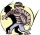The crime rate of a certain city is increasing by exactly 7% each year. If there were 600 crimes in the year 1990 and the crime rate remains constant each year, determine the approximate number of crimes in the year 2025.
2. The city 2Today lives 167000 citizens in city. How many citizens can we expect in 11 years if their annual increase is 1%?
3. BTC bubble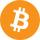One of the world's experts in bubbles -- Prof. Robert Shiller, a Yale economist -- said Bitcoin "was an amazing example of a bubble" back in 2014. If you exchange fiat US dollar \$100 to BTC in 2010, now in 2017 have value of 72.9 millions dollars. Find.A radioactive material loses 10% of its mass each year. What proportion will be left there after n=6 years?
5. Investment1000\$ is invested at 10% compound interest. What factor is the capital multiplied by each year? How much will be there after n=12 years?
6. Machine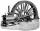Price of the new machine is € 62000. Every year is depreciated 15% of residual value. What will be the value of the machine after 3 years?
7. Enterprise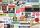The value of annual production enterprise is 7.1 million USD. The average annual growth rate is 1.5%. What will be value of production at the end of the fifth year?
8. Five membersWrite first 5 members geometric sequence and determine whether it is increasing or decreasing: a1 = 3 q = -2
9. Theorem proveWe want to prove the sentence: If the natural number n is divisible by six, then n is divisible by three. From what assumption we started?
10. A perineumA perineum string is 10% shorter than its original string. The first string is 24, what is the 9th string or term?
11. Geometric sequence 4It is given geometric sequence a3 = 7 and a12 = 3. Calculate s23 (= sum of the first 23 members of the sequence).
12. Geometric progression 2There is geometric sequence with a1=5.7 and quotient q=-2.5. Calculate a17.
13. Six termsFind the first six terms of the sequence a1 = -3, an = 2 * an-1
14. Tenth memberCalculate the tenth member of geometric sequence when given: a1=1/2 and q=2
15. Profit gainIf 5% more is gained by selling an article for Rs. 350 than by selling it for Rs. 340, the cost of the article is:
16. GP - 8 itemsDetermine the first eight members of a geometric progression if a9=512, q=2
17. Quotient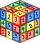Determine the quotient and the second member of the geometric progression where a3=10, a1+a2=-1,6 a1-a2=2,4.